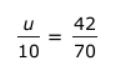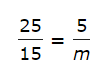# Learning About Ratios, Rates And, Proportions

This quiz contains various questions related to ratios, rates, and proportions. Various types of questions are added to the quiz to make the learning of such topics effective.

Start Quiz

William’s pencil box contains 3 pencils and 2 erasers. What is the ratio of erasers to pencils in William’s pencil box?

3:2

2:3

Are the ratios 4:5 and 20:25 equivalent?

yes

no

Are the ratios 3:7 and 21:25 equivalent?

yes

no

Select the ratio which is equivalent to 42:36?

7:4

14:12

12:15

Select the ratio which is equivalent to 22:11?

2:1

3:2

4:3

15 flowers in 5 vases = ##3## flowers per vase

3

12 kilometers in 3 hours = ##4## kilometers per hour

4

Solve for u in the proportion.5

6

7

8

Solve for m in the proportion.1

2

3

4

Sam walked a total of 24 kilometers by making 4 trips to school. After 8 trips to school, how many kilometers will Sam have walked in total? (Assuming directly proportional relation)

##48## kilometers

48
Quiz/Test Summary
Title: Learning About Ratios, Rates And, Proportions
Questions: 10
Contributed by: